Concepts

Class 9
Chapter 3 Class 9 - Atoms And Molecules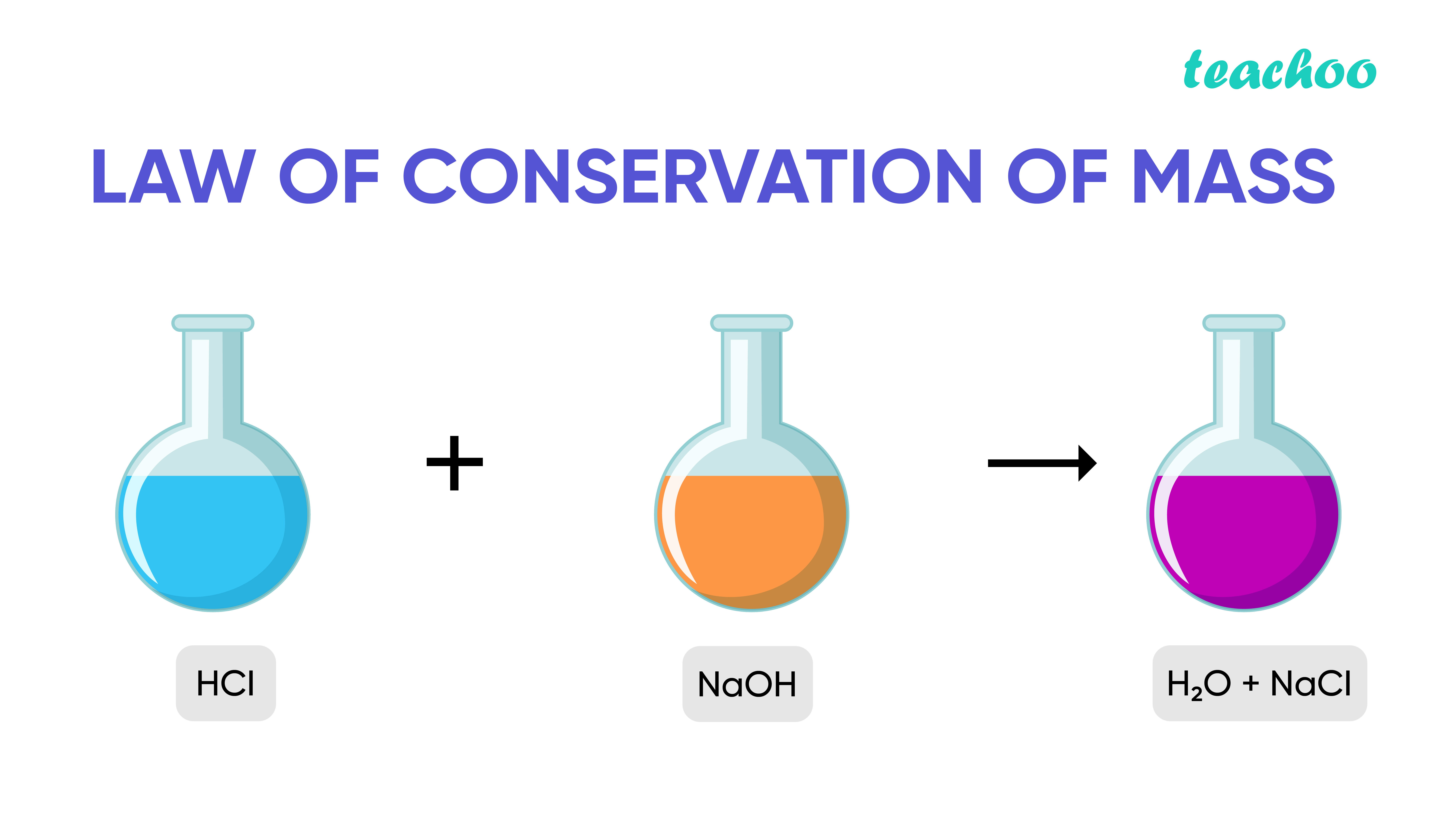Take a look at this image. What do you observe?

Here 2 substances react with each other to form 2 new substances .

This is called a Chemical Reaction .

Let us take a look at the different aspects of Chemical Reactions.

## What are Reactants and Products ?

During a chemical reaction, one or more substances react with each other (change) to produce one or more other substances

The substances which undergo chemical change are called Reactants

The substances formed after the reaction are called Products

Example 1:

When  Calcium Carbonate is heated,it produces Calcium oxide and Carbon Dioxide

Calcium Carbonate−−−−−−> Calcium Oxide +    Carbon Dioxide

1                 1st                 2nd

Reactant Product Product

Example 2:

Hydrogen and Oxygen combine to form Water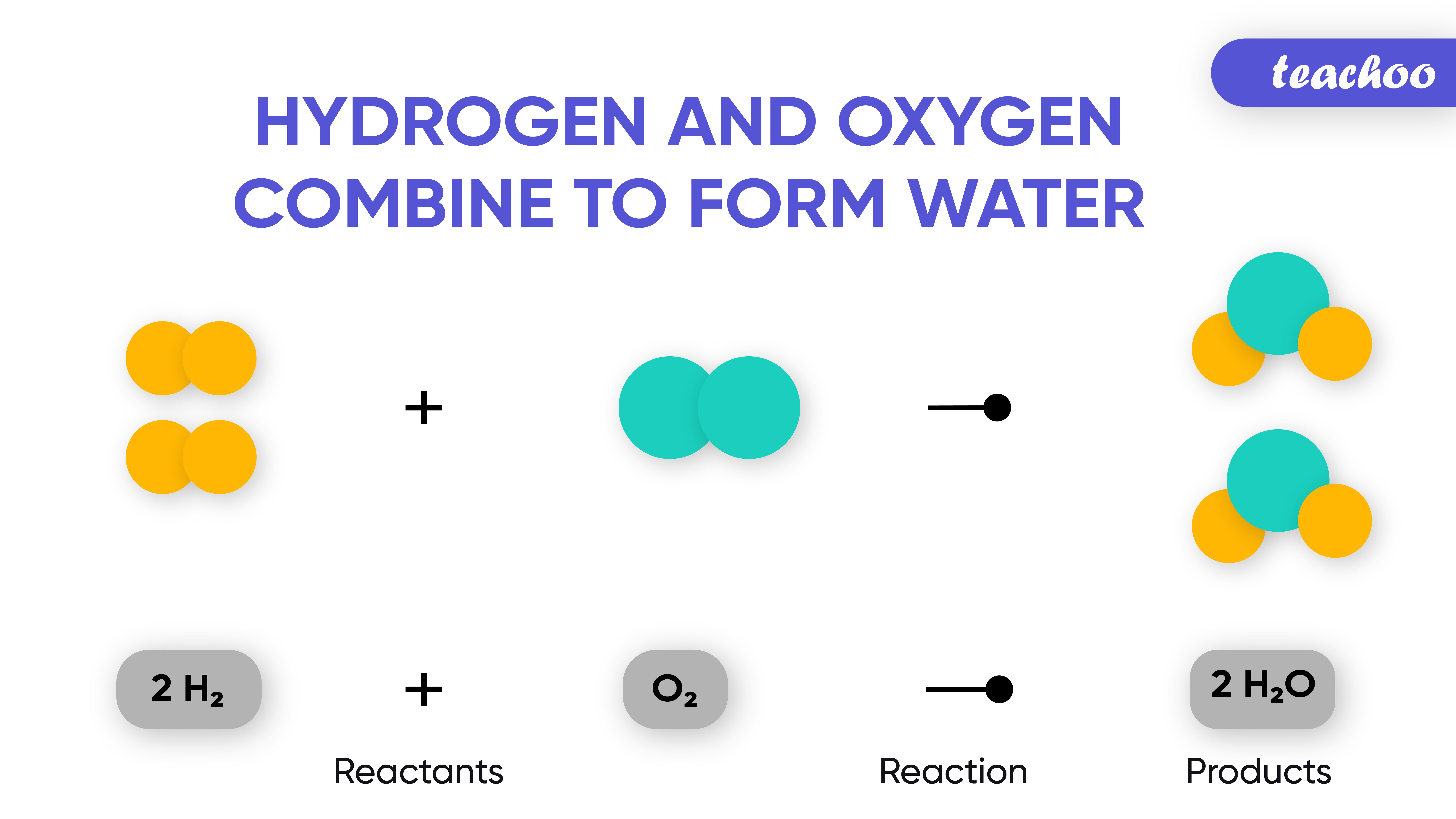## What is Law of Conservation of Mass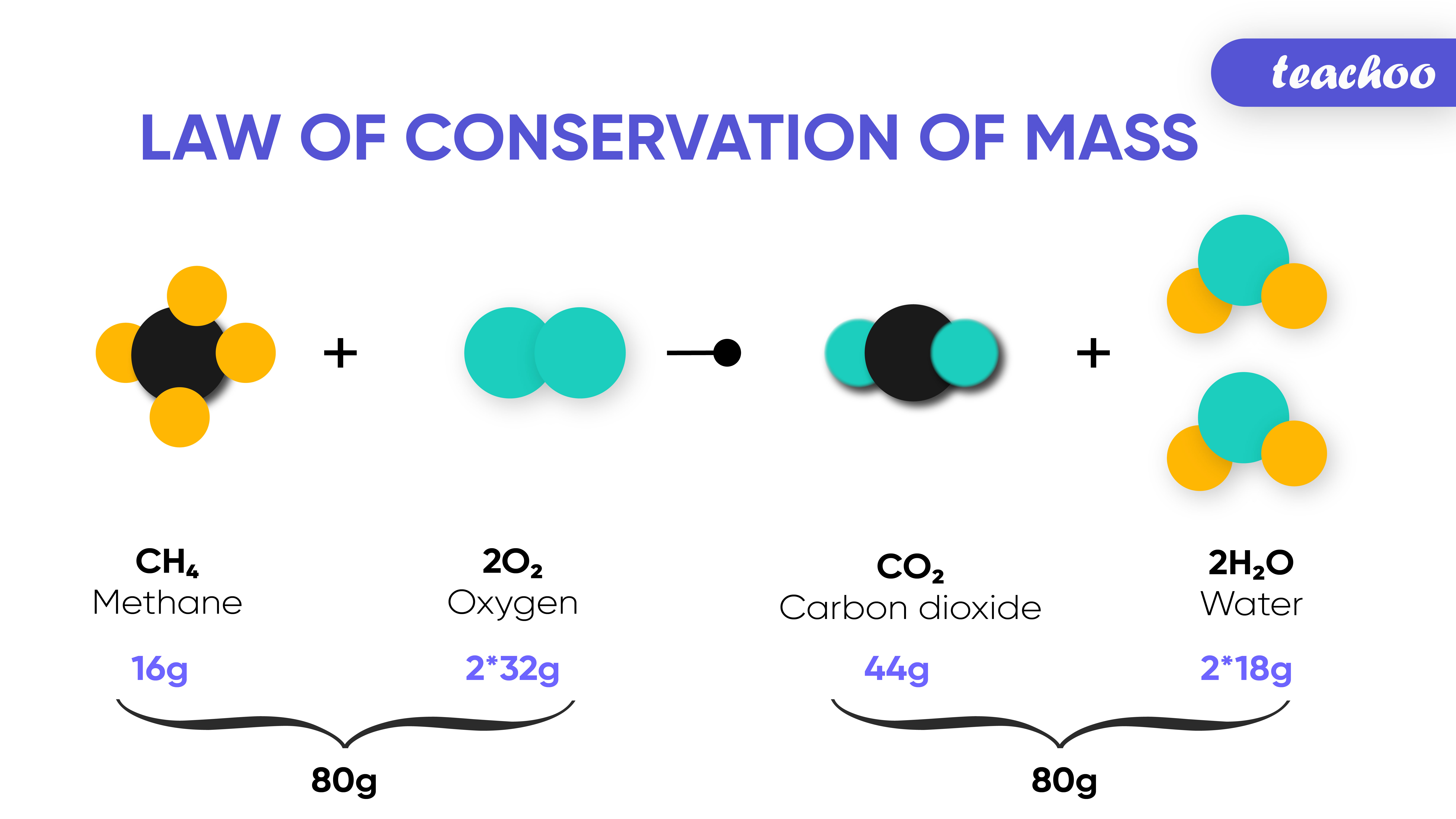What do you observe in the image above?

During a chemical reaction, the sum of the masses of the

reactants and products remain unchanged.

This is known as the Law of Conservation of Mass

As per this law ,

Matter can never be created nor destroyed

Total Mass of Reactants = Total Mass of Products

## Example 1

When 100 grams of calcium carbonate is heated,it produces 56 grams of  Calcium oxide and 44 grams of Carbon Dioxide

Calcium Carbonate −−−−−−> Calcium Oxide    +    Carbon Dioxide

1                 1st                 2nd

Reactant Product Product

(100 grams) 56 grams 44 grams

Total =56+44=100 grams

Total Mass of Reactants = Mass of Calcium Carbonate = 100 grams

Total Mass of Products = Mass of Calcium + Mass of Carbon Dioxide = 56 + 44 = 100 grams

Hence,

Total Mass of Reactants = Total Mass of Products

We can say that the sum of masses of reactants and products remain unchanged.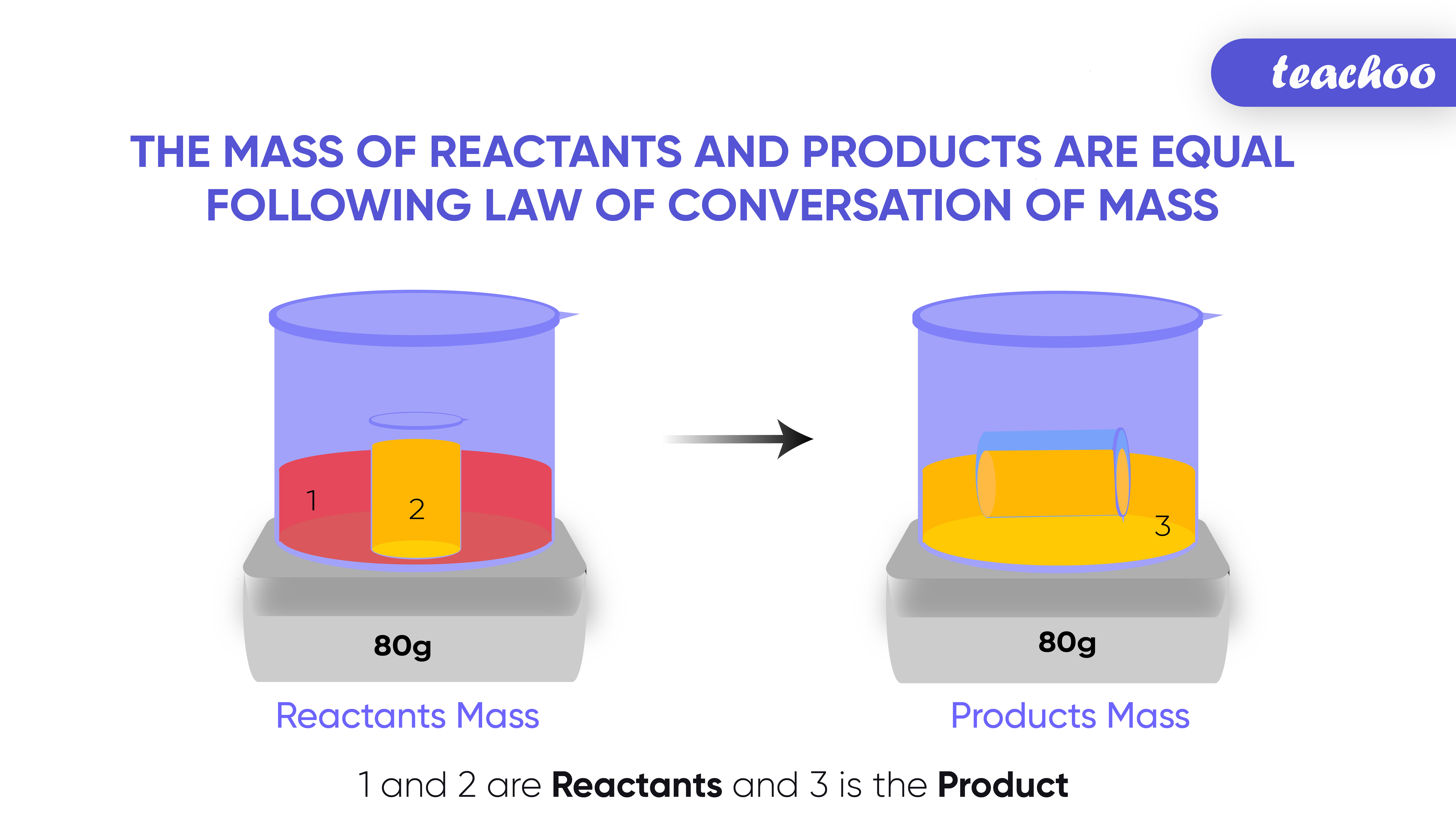Note:

Law of conservation of Mass can be applied to Physical Changes also

Example

If 100 grams of ice is melted, it produces 100 grams of water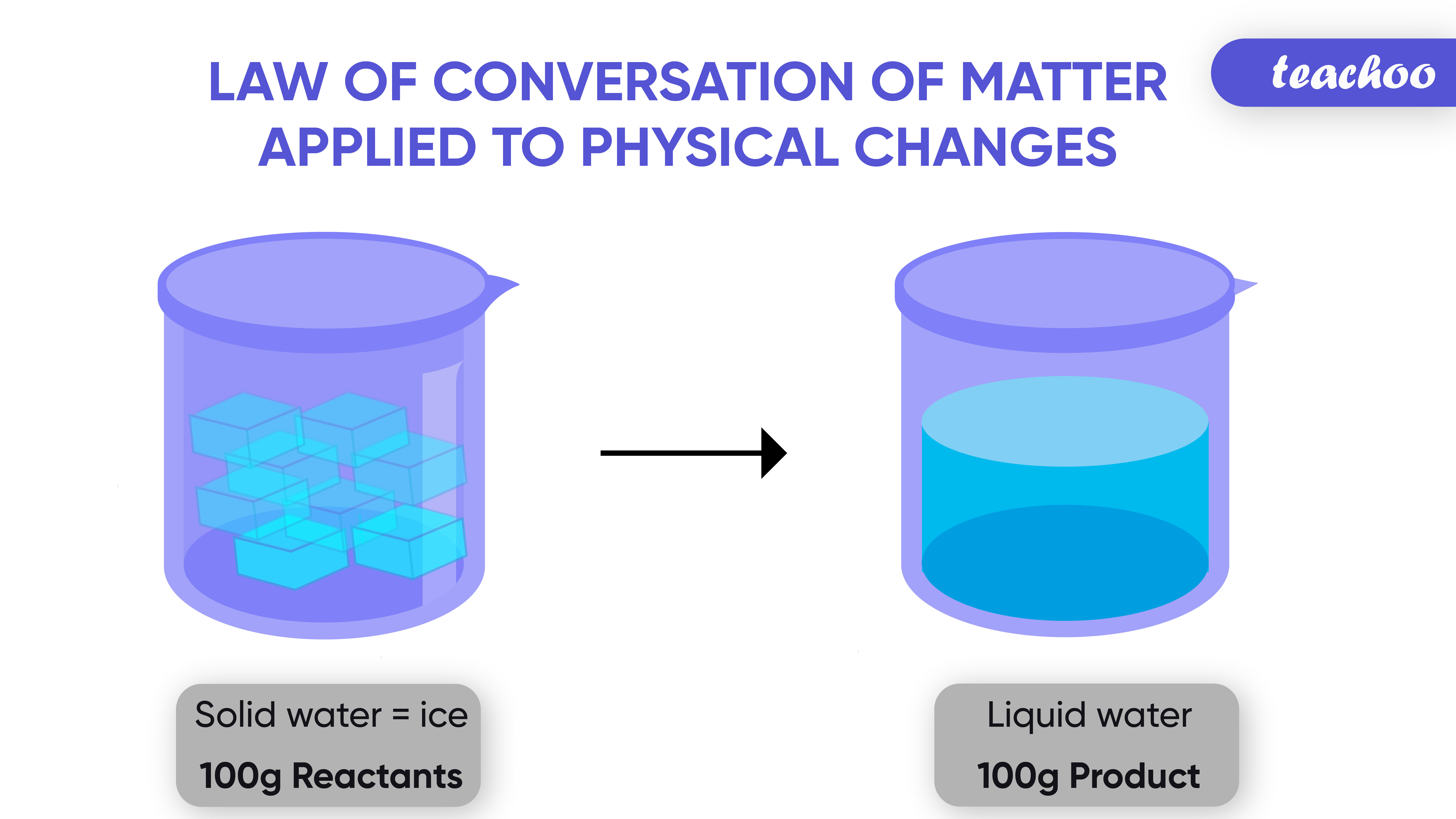Ice    −−−−−−−−−−−>    Water

100 grams 100 grams

1                 1

Reactant Product

Here also we can see that mass of initial and final stage of matter is the same

SAMPLE PROBLEM 1 LS PG 112

Example -

In text Quention - Page 32 Q1

NCERT Question - Q1

Get live Maths 1-on-1 Classs - Class 6 to 12